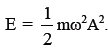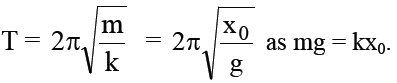# Concepts of Physics Ch-12 SHM (SA)

1. A person goes to bed at sharp 10:00 pm every day. Is it an example of periodic motion? If yes, what is the time period? If no, why?

Solution: No. As motion is a change in position of an object with respect to time or a reference point, it is not an example of periodic motion.

2. A particle executing simple harmonic motion comes to rest at the extreme positions. Is the resultant force on the particle zero at these positions according to Newton’s first law?

Solution: No. The resultant force on the particle is maximum at the extreme positions.

3. Can simple harmonic motion take place in a non-inertial frame? If yes, should the ratio of the force applied with the displacement be constant?

Solution: Yes. Simple harmonic motion can take place in a non-inertial frame. However, the ratio of the force applied to the displacement cannot be constant because a non-inertial frame has some acceleration with respect to the inertial frame. Therefore, a fictitious force should be added to explain the motion.

4. A particle executes simple harmonic motion. If you are told that its velocity at this instant is zero, can you say what is its displacement? If you are told that its velocity at this instant is maximum, can you say what is its displacement?

Solution: No, we cannot say anything from the given information. To determine the displacement of the particle using its velocity at any instant, its mean position has to be known.

5. A small creature moves with constant speed in a vertical circle on a bright day. Does its shadow formed by the sun on a horizontal plane move in a simple harmonic motion ?

Solution: Yes, its shadow on a horizontal plane moves in S.H.M. The projection of a uniform circular motion executes simple harmonic motion along its diameter (which is the shadow on the horizontal plane), with the mean position lying at the centre of the circle.

6. A particle executes simple harmonic motion. Let P be a point near the mean position and Q be a point near an extreme. The speed of the particle at P is larger than the speed at Q. Still the particle crosses P and Q equal number of times in a given time interval. Does it make you unhappy ?

Solution: No. It does not make me unhappy because the number of times a particle crosses the mean and extreme positions does not depend on the speed of the particle.

7. In measuring time period of a pendulum, it is advised to measure the time between consecutive passage through the mean position in the same direction. This is said to result in better accuracy than measuring time between  consecutive  passage  through  an  extreme position. Explain.

Solution: The mean position of a particle executing simple harmonic motion is fixed, whereas its extreme position keeps on changing. Therefore, when we use stop watch to measure the time between consecutive passages, we are certain about the mean position.

8. It is proposed to move a particle in simple harmonic motion on a rough horizontal surface by applying an external force along the line of motion. Sketch the graph of the applied force against the position of the particle. Note that the applied force has two values for a given position depending on whether the particle is moving in positive or negative direction.

Solution: Figure (a) shows the graph of the applied force against the position of the particle.9. Can the potential energy in a simple harmonic motion be negative? Will it be so if we choose zero potential energy at some point other than the mean position ?

Solution: No. it cannot be negative because the minimum potential energy of a particle executing S.H.M at mean position is zero. The potential energy increases in positive direction at the extreme position.

However, if we choose zero potential energy at some other point, say extreme position, the potential energy can be negative at the mean position.

10. The energy of a system in simple harmonic motion is given by,Which of the following two statements is more appropriate?

(A) The energy is increased because the amplitude is increased.

(B) The amplitude is increased because the energy is increased.

Solution: Statement B is more appropriate. The Amplitude is increased because energy is increased. Because, as energy is provided it will make a SHM with a larger amplitude to balance the energy.

11. A pendulum clock gives correct time at the equator. Will it gain or loose time as it is taken to the poles?

Solution: At pole, g is more so time period gets decreased hence clock gains time.

12. Can a pendulum clock be used in an earth-satellite?

Solution: No as g(effective) = 0

13. A hollow sphere filled with water is used as the bob of a pendulum. Assume that the equation for simple pendulum is valid with the distance between the point of suspension and centre of mass of the bob acting as the effective length of the pendulum. If water slowly leaks out of the bob, how will the time period vary?

Solution:First l increases and then it decreases.

14. A block of known mass is suspended from a fixed support through a light spring. Can you find the time period of vertical oscillation only by measuring the extension of the spring when the block is in equilibrium?

Solution: Yes.15. A platoon of soldiers marches on horizontal road in steps according to the sound of a marching band. The band is stopped and the soldiers are ordered to break the steps while crossing a bridge. Why?

Solution: Forced oscillation may break the bridge.

16. The force acting on a particle moving along X-axis is

F = -k(x- vt) where k is a positive constant. An observer moving at a constant velocity v along the X-axis looks at the particle. What kind of motion does he find for the particle?

Solution: Since he moves with constant velocity, he sees the same force. And this force is not that of SHM.

Announcements

Join our Online JEE Test Series for 499/- Only (Web + App) for 1 Year

Join our Online NEET Test Series for 499/- Only for 1 Year